# High School Math : Understanding Quadratic Equations

## Example Questions

1 3 Next →

### Example Question #3 : Understanding Quadratic Roots

Write an equation with the given roots: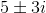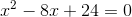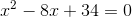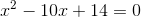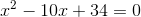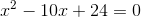Explanation:

To write an equation, find the sum and product of the roots. The sum is the negative coefficient of, and the product is the integer.

Sum: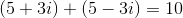Product: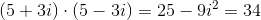Subtract the sum and add the product.

The equation is:### Example Question #4 : Understanding Quadratic Roots

Write an equation with the given roots: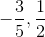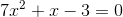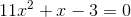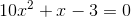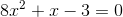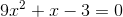Explanation:

To write an equation, find the sum and product of the roots. The sum is the negative coefficient of, and the product is the integer.

Sum: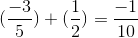Product: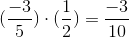Subtract the sum and add the product.

The equation is: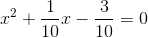Multiply the equation by:### Example Question #5 : Understanding Quadratic Roots

Write an equation with the given roots: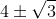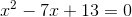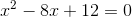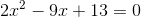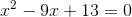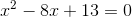Explanation:

To write an equation, find the sum and product of the roots. The sum is the negative coefficient of, and the product is the integer.

Sum: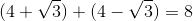Product: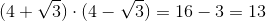Subtract the sum and add the product.

The equation is:1 3 Next →